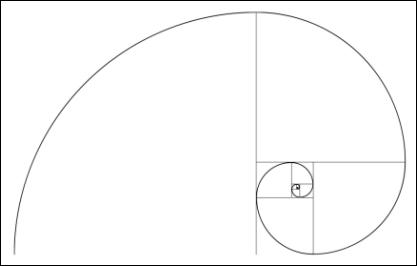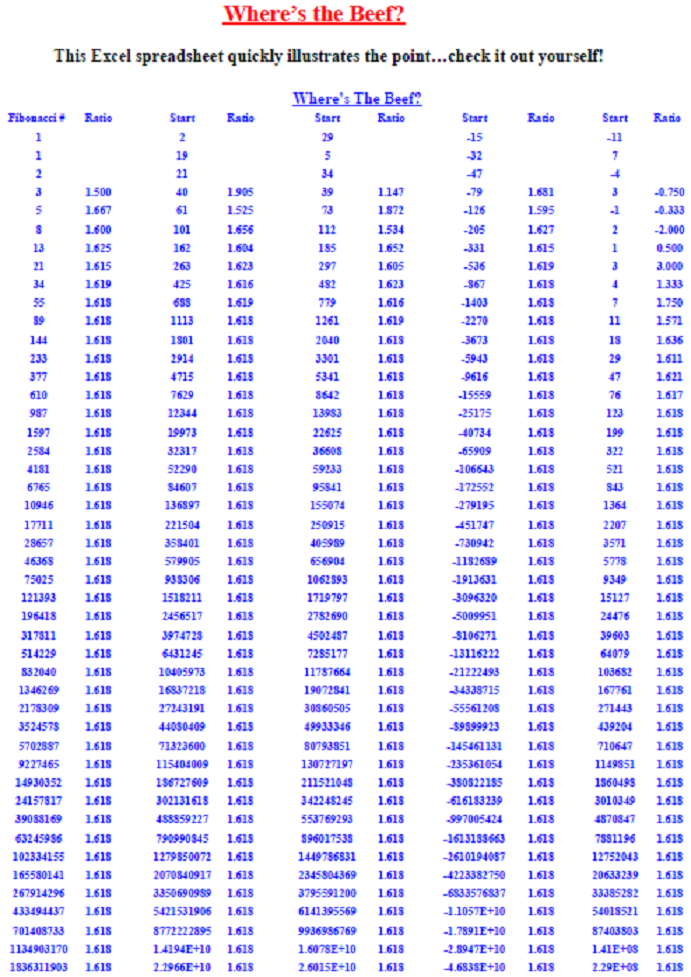# Junk Science; Junk Analysis!

|I don't think I have offended anyone in quite a while and feel I'm not doing my job if I don't try to periodically; so here goes!

Often a simple mathematical series of numbers can sometimes get misinterpreted (promoted) to be something magical. My personal favorite sequence is 6, 28, 496, 2520, 8128, and 24601. I'll explain them at the end of this article. Personally, I see no value in the actual numbers that make up the Fibonacci series; a series developed by an Italian mathematician (Fibonacci) in the thirteenth century to help understand the propagation of rabbits. First, I must say that I do value the ratio of the numbers that are expanded in a Fibonacci-like series (0, 1, 1, 2, 3, 5, 8, 13, 21, 34, 55, 89, ...). That ratio is 0.618 (and its reciprocal is 1.618), often called the golden ratio because of its wide occurrence in nature; almost always with a jaundiced eye. Here is a fact: the actual numbers in the

Fibonacci series have little to do with the ratio. Any two numbers expanded in the same manner will produce the same ‘golden' ratio. Here is a test: Try it with 2 and 19.  Add them together, and then add the total to the previous number just like in the Fibonacci series (2+19=21, 19+21=40, 21+40=61, etc.). Expand this until you get to four-digit numbers so that the accuracy will be acceptable (2, 19, 21, 40, 61, 101, 162, 263, 425, 688, 1113, 1801, 2914, 4716, ...).  The last two numbers in this sequence are the two numbers that I will use for this example: 2914 and 4716. Now divide the first number by the second number and you will get 0.618. This is exactly the same as with the value obtained using the Fibonacci series of numbers. So why did I pick 2 and 19 for this example? Hint: the second letter in the alphabet is B. What is the nineteenth letter? S. BS! And that is what numerology is all about. Table A shows the sequence using various starting numbers including my special selection of 2 and 19. The last column begins with a negative number.Table A

I can find no source that explains why the series of Fibonacci numbers begins at zero. If I were tasked with mathematically identifying the propagation of rabbits, I think I would at least have to begin the series at 2. The fact of the matter is that the series can begin anywhere, even negative numbers, as long as the expansion follows the correct formula. It is the ratio that is important, not the actual numbers in the series. So, when you hear someone say they are going to use a 34-day moving average because 34 is a Fibonacci number, you can immediately begin to doubt the rest of their analysis. Just so you know; the Fibonacci expansion of one plus the square root of five divided by 2 will work with any two numbers, even negative numbers. Sorry, no magic here, just numerology. As far as Elliott Wave theory goes, there are often so many complications and conditions introduced into using this type of analysis, that it is incapable of being proved wrong. Sometimes I think it gets adjusted more often than earnings estimates. However, it is always convincing to align the workings of the market with what appears to be pure mathematics. In the series of numbers introduced at the beginning of this section, 6, 28, 496, and 8128, are known as perfect numbers; this means the sum of their divisors (other than the number itself) is also equal to the number. For example: 6 = 1 + 2 + 3, and 28 = 1 + 2 + 4 + 7 + 14. I like 2520 because it is the smallest integer than is divisible by all integers from 1 to 10 inclusive. Finally, I like 24601 as it is the prisoner number of Jean Valjean from Victor Hugo's Les Miserables. Incidentally, 24601 has prime factors of 73 and 337. I like these numbers solely for their mathematical uniqueness; and like many number sequences, they have no use in technical market analysis. Possibly Keno! So why are people drawn to it so much? Probably because of its false potential for predicting the future and its cottage industry of numerology advocates.

I have known many successful traders and investors over the years. I do not know anyone who trades with real money that uses junk science like Fibonacci numbers. I put out articles like this because I see so many technical analysts that do not understand the tools they use; they accept them on face value. Hopefully, you can now see the problems with doing it that way.  So, were you offended by or appreciative of this article?

Dance with the Trend,

Greg MorrisAbout the author: has been a technical market analyst for over 45 years ranging from analysis software development, to website analysis and education, to money management. He has written four books: Candlestick Charting Explained (and its companion workbook), The Complete Guide to Market Breadth Indicators, and Investing with the Trend. A graduate of the Navy Fighter Weapons "Top Gun" School, Greg is a former Navy fighter pilot who flew F-4 Phantoms on the USS Independence.  He also holds a degree in Aerospace Engineering from the University of Texas. Greg has a long history of understanding market dynamics and portfolio management. Learn More